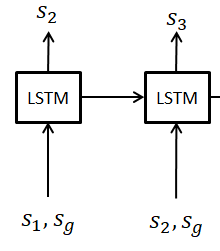# Implementation of RNN with an input which is a func of output at previous time-step

Hi, I got a question regarding the implementation of RNNs. In my implementation, the input to RNN at next time-step is basically the output of the RNN at previous timestep augmented with some external input. However, I was going through pytorch examples on RNNs (e.g., image captioning) and it seems we need to input the whole sequence of inputs at once into RNN as the input tensor should be of shape (seq_len, batch, input_size) which means my implementation wouldn’t be possible to implement. Is it correct or is there anyway to implement the architecture shown below?Unfortunately, it seems that we have to make a for loop, giving at each step the last output as a new input (one example here: http://pytorch.org/tutorials/intermediate/seq2seq_translation_tutorial.html).
Maybe there is already a way to make this without a hand-made loop, but I don’t know how.

One of my projects is to implement such a “self-feeding LSTM”, going into the underlying C code. I need to find the time.

Hi,

for epoch in range(args.num_epochs):
print “epoch” + str(epoch)
for i in range (0,int(dataset_train),args.batch_size):
# Forward, Backward and Optimize
for b in range(i,i+args.batch_size):
inp,sg = get_input(dataset[i]) # inp=torch.cat((s1,sg))
for l in range(0,len(targets)):
inp=to_var(inp)
outputs = RNN(inp)
inp=torch.cat((sg,outputs))
loss = criterion(outputs,targets[l])
loss.backward()
optimizer.step()

But it tells to give the input in the form (seq_length, batch, input_size).

You should make something like:

``````input = Variable(torch.Tensor([[sos]*batch_size])
hidden, cell = RNN.init_hidden_cell(batch_size)
for di in range(MAX_LENGTH):
output, (hidden,cell) = RNN(input, (hidden,cell))
loss += criterion(output, targets[di,:])
# for ex of a NLP output:
# contains the probabilities for each word IDs.
# The index of the probability is the word ID.
_, topi = torch.topk(output,1)
# topi = (batch*1)
input = torch.transpose(topi,0,1)
``````

And if you don’t use batchs, you can break the loop at the first “eos”.